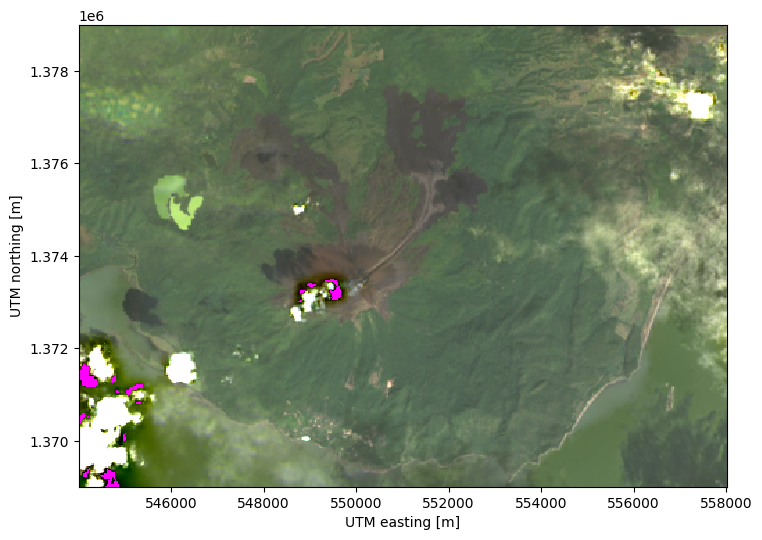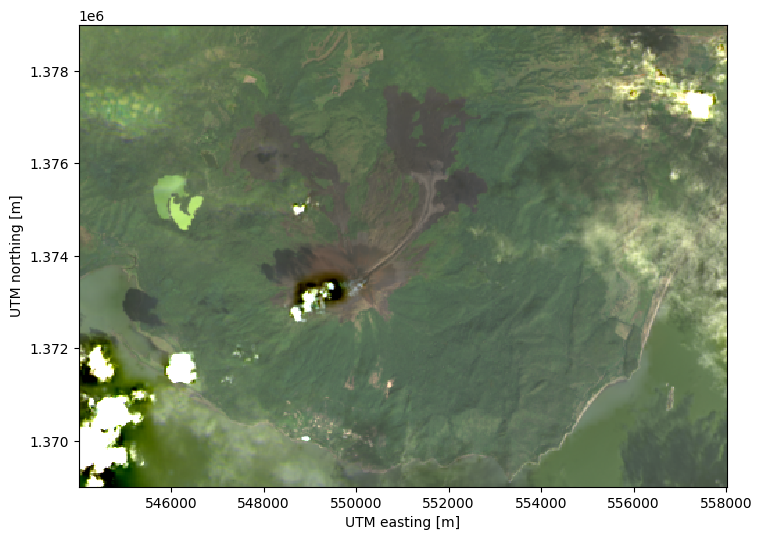# Filling missing values#

Landsat Level 2 data can sometimes contain missing values, particularly around bright clouds with dark shadows. These pixels will have a value of `numpy.nan` and can cause problems in other calculations. To fill them, we can use the values of neighboring pixels to interpolate the missing values with `xlandsat.interpolate_missing`.

Let’s use our sample scene of the December 2015 eruption of Momotombo volcano, Nicaragua, to demonstrate how it’s done.

First, we’ll import the required packages and load the sample scene:

```import xlandsat as xls
import matplotlib.pyplot as plt
import xarray as xr
import numpy as np

path = xls.datasets.fetch_momotombo()
```

Now we can plot an RGB composite to show some of the missing values. In order to highlight them, we’ll color the background of the plot in magenta so that the missing values don’t simply show up as white:

```# Make the composite and add it to the scene
rgb = xls.composite(scene, rescale_to=(0.04, 0.17))

fig, ax = plt.subplots(1, 1, figsize=(10, 6))

rgb.plot.imshow(ax=ax)

ax.set_facecolor("magenta")
ax.set_aspect("equal")

plt.show()
```We can fill these values with reasonable estimates using interpolation:

```scene_filled = xls.interpolate_missing(scene)

rgb_filled = xls.composite(scene_filled, rescale_to=(0.04, 0.17))

fig, ax = plt.subplots(1, 1, figsize=(10, 6))

rgb_filled.plot.imshow(ax=ax)

ax.set_facecolor("magenta")
ax.set_aspect("equal")

plt.show()
```The interpolated scene no longer contains the magenta patches!

Warning

This method works well when the missing data are only a few pixels or small patches. Large portions of the image missing cannot be filled in accurately by interpolation.